5 Functional description of the encoder

In this clause, the different functions of the encoder represented in figure 3 are described.

5.1 Pre‑processing (all modes)

Two pre‑processing functions are applied prior to the encoding process: high‑pass filtering and signal down‑scaling.

Down‑scaling consists of dividing the input by a factor of 2 to reduce the possibility of overflows in the fixed‑point implementation.

The high‑pass filter serves as a precaution against undesired low frequency components. A filter with a cut off frequency of 80 Hz is used, and it is given by: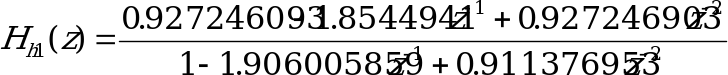. (4)

Down‑scaling and high‑pass filtering are combined by dividing the coefficients at the numerator of by 2.

5.2 Linear prediction analysis and quantization

12.2 kbit/s mode

Short‑term prediction, or linear prediction (LP), analysis is performed twice per speech frame using the auto‑correlation approach with 30 ms asymmetric windows. No lookahead is used in the auto‑correlation computation.

The auto‑correlations of windowed speech are converted to the LP coefficients using the Levinson‑Durbin algorithm. Then the LP coefficients are transformed to the Line Spectral Pair (LSP) domain for quantization and interpolation purposes. The interpolated quantified and unquantized filter coefficients are converted back to the LP filter coefficients (to construct the synthesis and weighting filters at each subframe).

10.2, 7.95, 7.40, 6.70, 5.90, 5.15, 4.75 kbit/s modes

Short‑term prediction, or linear prediction (LP), analysis is performed once per speech frame using the auto‑correlation approach with 30 ms asymmetric windows. A lookahead of 40 samples (5 ms) is used in the auto‑correlation computation.

The auto‑correlations of windowed speech are converted to the LP coefficients using the Levinson‑Durbin algorithm. Then the LP coefficients are transformed to the Line Spectral Pair (LSP) domain for quantization and interpolation purposes. The interpolated quantified and unquantized filter coefficients are converted back to the LP filter coefficients (to construct the synthesis and weighting filters at each subframe).

5.2.1 Windowing and auto‑correlation computation

12.2 kbit/s mode

LP analysis is performed twice per frame using two different asymmetric windows. The first window has its weight concentrated at the second subframe and it consists of two halves of Hamming windows with different sizes. The window is given by:

(5)

The values and are used. The second window has its weight concentrated at the fourth subframe and it consists of two parts: the first part is half a Hamming window and the second part is a quarter of a cosine function cycle. The window is given by:

(6)

where the values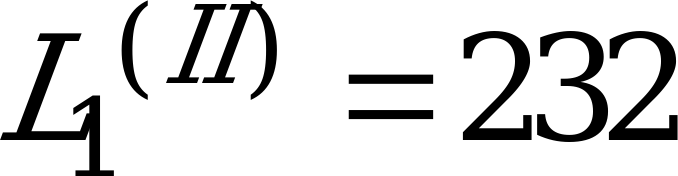and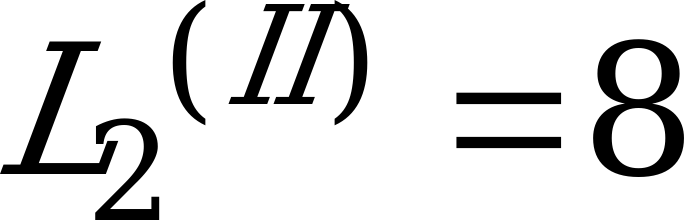are used.

Note that both LP analyses are performed on the same set of speech samples. The windows are applied to 80 samples from past speech frame in addition to the 160 samples of the present speech frame. No samples from future frames are used (no lookahead). A diagram of the two LP analysis windows is depicted below.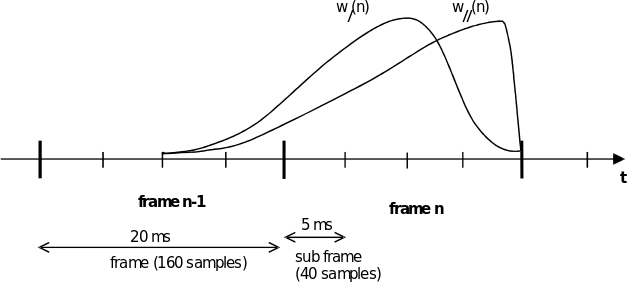Figure 1: LP analysis windows

The auto‑correlations of the windowed speech , are computed by:

(7)

and a 60 Hz bandwidth expansion is used by lag windowing the auto‑correlations using the window:

, (8)

where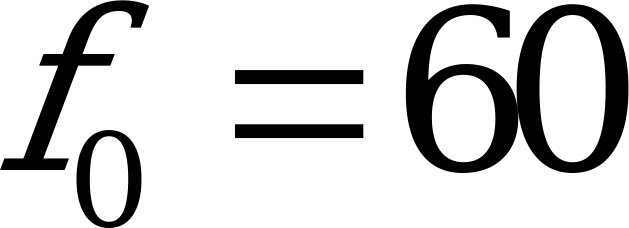Hz is the bandwidth expansion and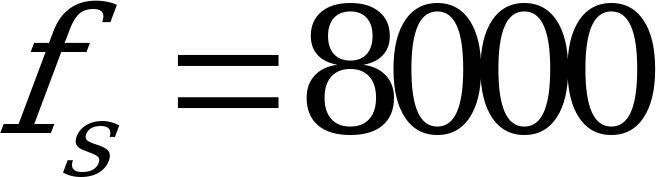Hz is the sampling frequency. Further, is multiplied by the white noise correction factor 1.0001 which is equivalent to adding a noise floor at ‑40 dB.

10.2, 7.95, 7.40, 6.70, 5.90, 5.15, 4.75 kbit/s modes

LP analysis is performed once per frame using an asymmetric window. The window has its weight concentrated at the fourth subframe and it consists of two parts: the first part is half a Hamming window and the second part is a quarter of a cosine function cycle. The window is given by equation (6) where the valuesand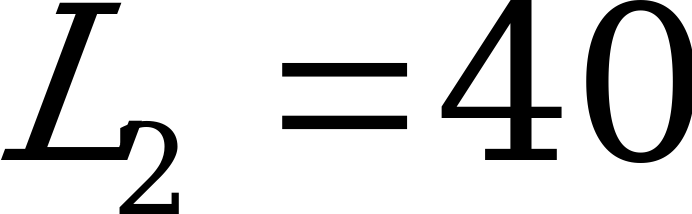are used.

The auto‑correlations of the windowed speech , are computed by equation (7) and a 60 Hz bandwidth expansion is used by lag windowing the auto‑correlations using the window of equation (8). Further, is multiplied by the white noise correction factor 1.0001 which is equivalent to adding a noise floor at ‑40 dB.

5.2.2 Levinson‑Durbin algorithm (all modes)

The modified auto‑correlations and are used to obtain the direct form LP filter coefficients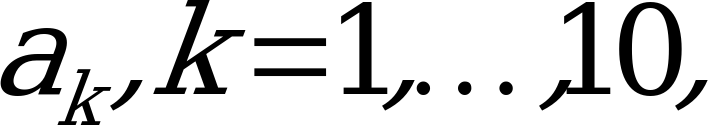by solving the set of equations.

(9)

The set of equations in (9) is solved using the Levinson‑Durbin algorithm. This algorithm uses the following recursion:

The final solution is given as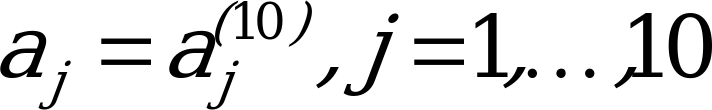.

The LP filter coefficients are converted to the line spectral pair (LSP) representation for quantization and interpolation purposes. The conversions to the LSP domain and back to the LP filter coefficient domain are described in the next clause.

5.2.3 LP to LSP conversion (all modes)

The LP filter coefficients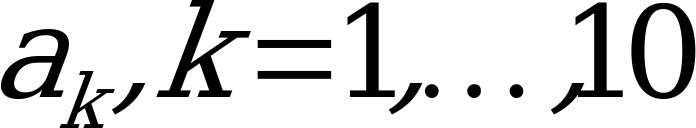, are converted to the line spectral pair (LSP) representation for quantization and interpolation purposes. For a 10th order LP filter, the LSPs are defined as the roots of the sum and difference polynomials:

(10)

and

, (11)

respectively. The polynomial and are symmetric and anti‑symmetric, respectively. It can be proven that all roots of these polynomials are on the unit circle and they alternate each other. has a root () and has a root (). To eliminate these two roots, we define the new polynomials:

(12)

and

(13)

Each polynomial has 5 conjugate roots on the unit circle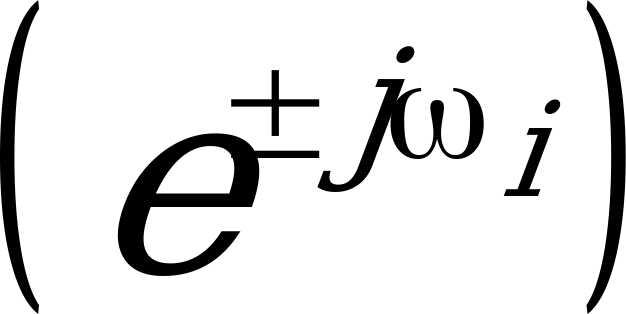, therefore, the polynomials can be written as

(14)

and

, (15)

where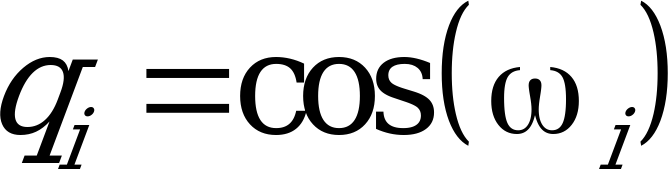with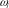being the line spectral frequencies (LSF) and they satisfy the ordering property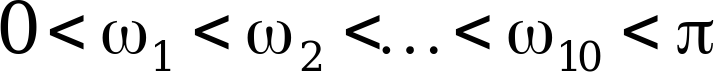. We refer to as the LSPs in the cosine domain.

Since both polynomials and are symmetric only the first 5 coefficients of each polynomial need to be computed. The coefficients of these polynomials are found by the recursive relations (for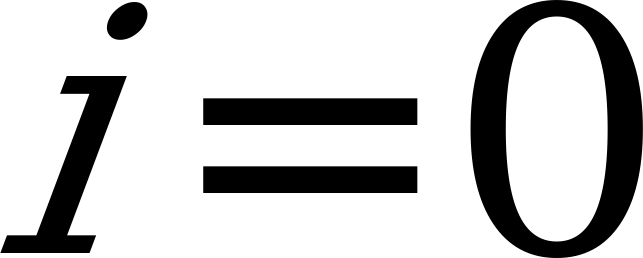to 4):

(16)

whereis the predictor order.

The LSPs are found by evaluating the polynomials and at 60 points equally spaced between 0 andand checking for sign changes. A sign change signifies the existence of a root and the sign change interval is then divided 4 times to better track the root. The Chebyshev polynomials are used to evaluate and . In this method the roots are found directly in the cosine domain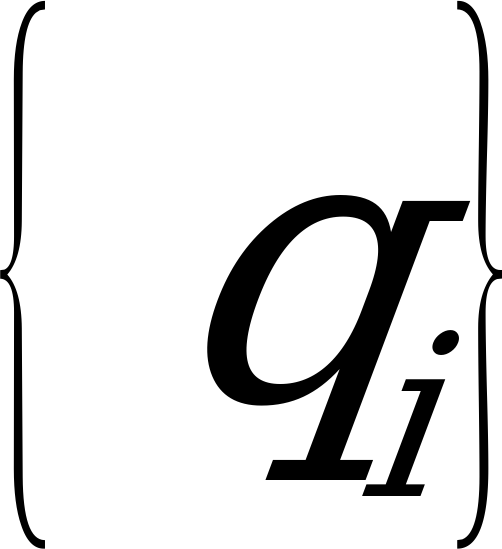. The polynomials or evaluated at can be written as:

,

with:

, (17)

where is theth order Chebyshev polynomial, and are the coefficients of either or , computed using the equations in (16). The polynomial is evaluated at a certain value of using the recursive relation:

with initial values and The details of the Chebyshev polynomial evaluation method are found in P. Kabal and R.P. Ramachandran .

5.2.4 LSP to LP conversion (all modes)

Once the LSPs are quantified and interpolated, they are converted back to the LP coefficient domain . The conversion to the LP domain is done as follows. The coefficients of or are found by expanding equations (14) and (15) knowing the quantified and interpolated LSPs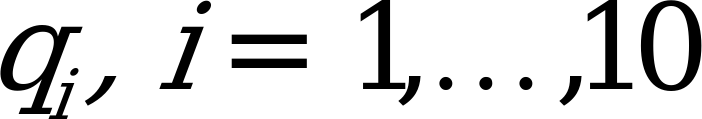. The following recursive relation is used to compute :

with initial values and . The coefficients are computed similarly by replacing by .

Once the coefficients and are found, and are multiplied by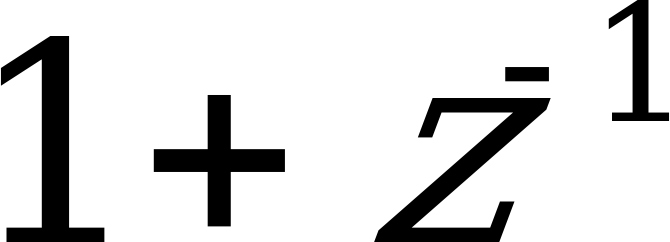and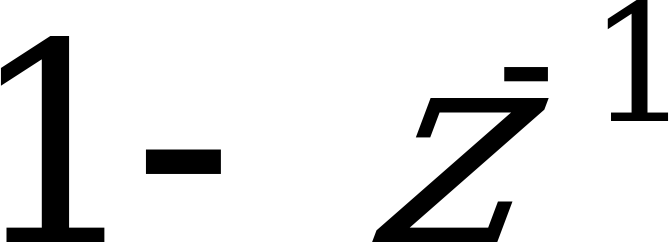, respectively, to obtain and ; that is:

. (18)

Finally the LP coefficients are found by:

. (19)

This is directly derived from the relation , and considering the fact that and are symmetric and anti‑symmetric polynomials, respectively.

5.2.5 Quantization of the LSP coefficients

12.2 kbit/s mode

The two sets of LP filter coefficients per frame are quantified using the LSP representation in the frequency domain; that is:

(20)

where are the line spectral frequencies (LSF) in Hz [0,4000] and is the sampling frequency. The LSF vector is given by , with t denoting transpose.

A 1st order MA prediction is applied, and the two residual LSF vectors are jointly quantified using split matrix quantization (SMQ). The prediction and quantization are performed as follows. Let and denote the mean‑removed LSF vectors at frame . The prediction residual vectors and are given by:

(21)

where is the predicted LSF vector at frame . First order moving‑average (MA) prediction is used where:

, (22)

where is the quantified second residual vector at the past frame.

The two LSF residual vectors and are jointly quantified using split matrix quantization (SMQ). The matrix is split into 5 submatrices of dimension 2 x 2 (two elements from each vector). For example, the first submatrix consists of the elements , , , and . The 5 submatrices are quantified with 7, 8, 8+1, 8, and 6 bits, respectively. The third submatrix uses a 256‑entry signed codebook (8‑bit index plus 1‑bit sign).

A weighted LSP distortion measure is used in the quantization process. In general, for an input LSP vector and a quantified vector at index , , the quantization is performed by finding the index which minimizes:

(23)

The weighting factors , are given by

(24)

where with and . Here, two sets of weighting coefficients are computed for the two LSF vectors. In the quantization of each submatrix, two weighting coefficients from each set are used with their corresponding LSFs.

10.2, 7.95, 7.40, 6.70, 5.90, 5.15, 4.75 kbit/s modes

The set of LP filter coefficients per frame is quantified using the LSP representation in the frequency domain using equation (20).

A 1st order MA prediction is applied, and the residual LSF vector is quantified using split vector quantization. The prediction and quantization are performed as follows. Let denote the mean‑removed LSF vectors at frame . The prediction residual vectors is given by:

(25)

where is the predicted LSF vector at frame . First order moving‑average (MA) prediction is used where:

, (26)

where is the quantified residual vector at the past frame and is the prediction factor for the jth LSF.

The LSF residual vectors is quantified using split vector quantization. The vector is split into 3 subvectors of dimension 3, 3, and 4. The 3 subvectors are quantified with 7-9 bits according to table 2.

Table 2: Bit allocation split vector quantization of LSF residual vector

 Mode Subvector 1 Subvector 2 Subvector 3 10.2 kbit/s 8 9 9 7.95 kbit/s 9 9 9 7.40 kbit/s 8 9 9 6.70 kbit/s 8 9 9 5.90 kbit/s 8 9 9 5.15 kbit/s 8 8 7 4.75 kbit/s 8 8 7

The weighted LSP distortion measure of equation (23) with the weighting of equation (24) is used in the quantization process.

5.2.6 Interpolation of the LSPs

12.2 kbit/s mode

The two sets of quantified (and unquantized) LP parameters are used for the second and fourth subframes whereas the first and third subframes use a linear interpolation of the parameters in the adjacent subframes. The interpolation is performed on the LSPs in the domain. Let be the LSP vector at the 4th subframe of the present frame , be the LSP vector at the 2nd subframe of the present frame , and the LSP vector at the 4th subframe of the past frame . The interpolated LSP vectors at the 1st and 3rd subframes are given by:

(27)

The interpolated LSP vectors are used to compute a different LP filter at each subframe (both quantified and unquantized coefficients) using the LSP to LP conversion method described in subclause 5.2.4.

10.2, 7.95, 7.40, 6.70, 5.90, 5.15, 4.75 kbit/s modes

The set of quantified (and unquantized) LP parameters is used for the fourth subframe whereas the first, second, and third subframes use a linear interpolation of the parameters in the adjacent subframes. The interpolation is performed on the LSPs in the domain. The interpolated LSP vectors at the 1st, 2nd, and 3rd subframes are given by:

(28)

The interpolated LSP vectors are used to compute a different LP filter at each subframe (both quantified and unquantized coefficients) using the LSP to LP conversion method described in subclause 5.2.4.

5.2.7 Monitoring resonance in the LPC spectrum (all modes)

Resonances in the LPC filter are monitored to detect possible problem areas where divergence between the adaptive codebook memories in the encoder and the decoder could cause unstable filters in areas with highly correlated continuos signals. Typically, this divergence is due to channel errors.

The monitoring of resonance signals is performed using unquantized LSPs . The LSPs are available after the LP to LSP conversion in section 5.2.3. The algorithm utilises the fact that LSPs are closely located at a peak in the spectrum. First, two distances, and , are calculated in two different regions, defined as , and .

Either of these two minimum distance conditions must be fulfilled to classify the frame as a resonance frame and increase the resonance counter.

is a fixed threshold while the second one is depending on according to:

12 consecutive resonance frames are needed to indicate possible problem conditions, otherwise the LSP_flag is cleared.

5.3 Open‑loop pitch analysis

Open‑loop pitch analysis is performed in order to simplify the pitch analysis and confine the closed‑loop pitch search to a small number of lags around the open‑loop estimated lags.

Open‑loop pitch estimation is based on the weighted speech signal which is obtained by filtering the input speech signal through the weighting filter . That is, in a subframe of size , the weighted speech is given by:

(29)

12.2 kbit/s mode

Open‑loop pitch analysis is performed twice per frame (each 10 ms) to find two estimates of the pitch lag in each frame.

Open‑loop pitch analysis is performed as follows. In the first step, 3 maxima of the correlation:

(30)

are found in the three ranges:

The retained maxima , are normalized by dividing by , respectively. The normalized maxima and corresponding delays are denoted by . The winner, , among the three normalized correlations is selected by favouring the delays with the values in the lower range. This is performed by weighting the normalized correlations corresponding to the longer delays. The best open‑loop delay is determined as follows:

This procedure of dividing the delay range into 3 clauses and favouring the lower clauses is used to avoid choosing pitch multiples.

10.2 kbit/s mode

Open-loop pitch analysis is performed twice per frame (every 10 ms) to find two estimates of the pitch lag in each frame.

The open-loop pitch analysis is performed as follows. First, the correlation of weighted speech is determined for each pitch lag value d by:

, (31)

where is a weighting function. The estimated pitch-lag is the delay that maximises the weighted correlation function . The weighting emphasises lower pitch lag values reducing the likelihood of selecting a multiple of the correct delay. The weighting function consists of two parts: a low pitch lag emphasis function, , and a previous frame lag neighbouring emphasis function, :

. (32)

The low pitch lag emphasis function is a given by:

(33)

where is defined by a table in the fixed point computational computational description (ANSI-C code) in GSM 06.73 . The previous frame lag neighbouring emphasis function depends on the pitch lag of previous speech frames:

(34)

where , is the median filtered pitch lag of 5 previous voiced speech half-frames, and v is an adaptive parameter. If the frame is classified as voiced by having the open-loop gain , the v-value is set to 1.0 for the next frame. Otherwise, the v-value is updated by . The open loop gain is given by:

(35)

where is the pitch delay that maximizes . The median filter is updated only during voiced speech frames. The weighting depends on the reliability of the old pitch lags. If previous frames have contained unvoiced speech or silence, the weighting is attenuated through the parameter v.

7.95, 7.40, 6.70, 5.90 kbit/s modes

Open‑loop pitch analysis is performed twice per frame (each 10 ms) to find two estimates of the pitch lag in each frame.

Open‑loop pitch analysis is performed as follows. In the first step, 3 maxima of the correlation in equation (30) are found in the three ranges:

The retained maxima , are normalized by dividing by , respectively. The normalized maxima and corresponding delays are denoted by . The winner, , among the three normalized correlations is selected by favouring the delays with the values in the lower range. This is performed by weighting the normalized correlations corresponding to the longer delays. The best open‑loop delay is determined as follows:

This procedure of dividing the delay range into 3 clauses and favouring the lower clauses is used to avoid choosing pitch multiples.

5.15, 4.75 kbit/s modes

Open‑loop pitch analysis is performed once per frame (each 20 ms) to find an estimate of the pitch lag in each frame.

Open‑loop pitch analysis is performed as follows. In the first step, 3 maxima of the correlation in equation (30) are found in the three ranges:

The retained maxima , are normalized by dividing by , respectively. The normalized maxima and corresponding delays are denoted by . The winner, , among the three normalized correlations is selected by favouring the delays with the values in the lower range. This is performed by weighting the normalized correlations corresponding to the longer delays. The best open‑loop delay is determined as follows:

This procedure of dividing the delay range into 3 clauses and favouring the lower clauses is used to avoid choosing pitch multiples.

5.4 Impulse response computation (all modes)

The impulse response, , of the weighted synthesis filter is computed each subframe. This impulse response is needed for the search of adaptive and fixed codebooks. The impulse response is computed by filtering the vector of coefficients of the filter extended by zeros through the two filters and .

5.5 Target signal computation (all modes)

The target signal for adaptive codebook search is usually computed by subtracting the zero input response of the weighted synthesis filter from the weighted speech signal . This is performed on a subframe basis.

An equivalent procedure for computing the target signal, which is used in this standard, is the filtering of the LP residual signal through the combination of synthesis filter and the weighting filter . After determining the excitation for the subframe, the initial states of these filters are updated by filtering the difference between the LP residual and excitation. The memory update of these filters is explained in subclause 5.9.

The residual signal which is needed for finding the target vector is also used in the adaptive codebook search to extend the past excitation buffer. This simplifies the adaptive codebook search procedure for delays less than the subframe size of 40 as will be explained in the next clause. The LP residual is given by:

(36)

Adaptive codebook search is performed on a subframe basis. It consists of performing closed‑loop pitch search, and then computing the adaptive codevector by interpolating the past excitation at the selected fractional pitch lag.

The adaptive codebook parameters (or pitch parameters) are the delay and gain of the pitch filter. In the adaptive codebook approach for implementing the pitch filter, the excitation is repeated for delays less than the subframe length. In the search stage, the excitation is extended by the LP residual to simplify the closed‑loop search.

12.2 kbit/s mode

In the first and third subframes, a fractional pitch delay is used with resolutions: 1/6 in the range and integers only in the range [95, 143]. For the second and fourth subframes, a pitch resolution of 1/6 is always used in the range , where is nearest integer to the fractional pitch lag of the previous (1st or 3rd) subframe, bounded by 18…143.

Closed‑loop pitch analysis is performed around the open‑loop pitch estimates on a subframe basis. In the first (and third) subframe the range , bounded by 18…143, is searched. For the other subframes, closed‑loop pitch analysis is performed around the integer pitch selected in the previous subframe, as described above. The pitch delay is encoded with 9 bits in the first and third subframes and the relative delay of the other subframes is encoded with 6 bits.

The closed‑loop pitch search is performed by minimizing the mean‑square weighted error between the original and synthesized speech. This is achieved by maximizing the term:

(37)

where is the target signal and is the past filtered excitation at delay (past excitation convolved with ). Note that the search range is limited around the open‑loop pitch as explained earlier.

The convolution is computed for the first delay in the searched range, and for the other delays in the search range , it is updated using the recursive relation:

, (38)

where , is the excitation buffer. Note that in search stage, the samples, are not known, and they are needed for pitch delays less than 40. To simplify the search, the LP residual is copied to in order to make the relation in equation (38) valid for all delays.

Once the optimum integer pitch delay is determined, the fractions from –3/6 to 3/6 with a step of 1/6 around that integer are tested. The fractional pitch search is performed by interpolating the normalized correlation in equation (37) and searching for its maximum. The interpolation is performed using an FIR filter based on a Hamming windowed function truncated at  23 and padded with zeros at  24 (). The filter has its cut‑off frequency (‑3 dB) at 3 600 Hz in the over‑sampled domain. The interpolated values of for the fractions –3/6 to 3/6 are obtained using the interpolation formula:

(39)

where corresponds to the fractions 0, 1/6, 2/6, 3/6, -2/6, and –1/6, respectively. Note that it is necessary to compute the correlation terms in equation (37) using a range to allow for the proper interpolation.

Once the fractional pitch lag is determined, the adaptive codebook vector is computed by interpolating the past excitation signal at the given integer delay and phase (fraction) :

(40)

The interpolation filter is based on a Hamming windowed function truncated at  59 and padded with zeros at  60 (). The filter has a cut‑off frequency (‑3 dB) at 3 600 Hz in the over‑sampled domain.

The adaptive codebook gain is then found by: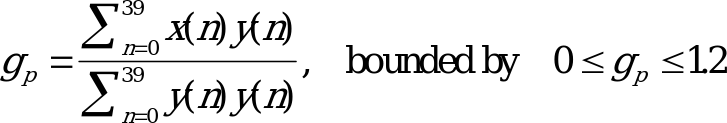(41)

where is the filtered adaptive codebook vector (zero state response of to ).

The computed adaptive codebook gain is quantified using 4‑bit non‑uniform scalar quantization in the range [0.0,1.2].

7.95 kbit/s mode

In the first and third subframes, a fractional pitch delay is used with resolutions: 1/3 in the range and integers only in the range [85, 143]. For the second and fourth subframes, a pitch resolution of 1/3 is always used in the range , where is nearest integer to the fractional pitch lag of the previous (1st or 3rd) subframe, bounded by 20…143.

Closed‑loop pitch analysis is performed around the open‑loop pitch estimates on a subframe basis. In the first (and third) subframe the range , bounded by 20…143, is searched. For the other subframes, closed‑loop pitch analysis is performed around the integer pitch selected in the previous subframe, as described above. The pitch delay is encoded with 8 bits in the first and third subframes and the relative delay of the other subframes is encoded with 6 bits.

The closed‑loop pitch search is performed by minimizing the mean‑square weighted error between the original and synthesized speech. This is achieved by maximizing the term of equation (37). Note that the search range is limited around the open‑loop pitch as explained earlier.

The convolution is computed for the first delay in the searched range, and for the other delays in the search range , it is updated using the recursive relation of equation (38).

Once the optimum integer pitch delay is determined, the fractions from –2/3 to 2/3 with a step of 1/3 around that integer are tested. The fractional pitch search is performed by interpolatingthe normalized correlation in equation (37) and searching for its maximum. Once the fractional pitch lag is determined, the adaptive codebook vector is computed by interpolating the past excitation signal at the given integer delay and phase (fraction). The interpolation is performed using two FIR filters (Hamming windowed sinc functions); one for interpolating the term in equation (37) with the sinc truncated at  11 and the other for interpolating the past excitation with the sinc truncated at  29. The filters have their cut‑off frequency (‑3 dB) at 3 600 Hz in the over‑sampled domain.

The adaptive codebook gain is then found as in equation (41).

The computed adaptive codebook gain is quantified using 4‑bit non‑uniform scalar quantization as described in section 5.8.

10.2, 7.40 kbit/s mode

In the first and third subframes, a fractional pitch delay is used with resolutions: 1/3 in the range and integers only in the range [85, 143]. For the second and fourth subframes, a pitch resolution of 1/3 is always used in the range , where is nearest integer to the fractional pitch lag of the previous (1st or 3rd) subframe, bounded by 20…143.

Closed‑loop pitch analysis is performed around the open‑loop pitch estimates on a subframe basis. In the first (and third) subframe the range , bounded by 20…143, is searched. For the other subframes, closed‑loop pitch analysis is performed around the integer pitch selected in the previous subframe, as described above. The pitch delay is encoded with 8 bits in the first and third subframes and the relative delay of the other subframes is encoded with 5 bits.

The closed‑loop pitch search is performed by minimizing the mean‑square weighted error between the original and synthesized speech. This is achieved by maximizing the term of equation (37). Note that the search range is limited around the open‑loop pitch as explained earlier.

The convolution is computed for the first delay in the searched range, and for the other delays in the search range , it is updated using the recursive relation of equation (38).

Once the optimum integer pitch delay is determined, the fractions from –2/3 to 2/3 with a step of 1/3 around that integer are tested. The fractional pitch search is performed by interpolatingthe normalized correlation in equation (37) and searching for its maximum. Once the fractional pitch lag is determined, the adaptive codebook vector is computed by interpolating the past excitation signal at the given integer delay and phase (fraction). The interpolation is performed using two FIR filters (Hamming windowed sinc functions); one for interpolating the term in equation (37) with the sinc truncated at  11 and the other for interpolating the past excitation with the sinc truncated at  29. The filters have their cut‑off frequency (‑3 dB) at 3 600 Hz in the over‑sampled domain.

The adaptive codebook gain is then found as in equation (41).

The computed adaptive codebook gain (and the fixed codebook gain) is quantified using 7‑bit non‑uniform vector quantization as described in section 5.8.

6.70, 5.90 kbit/s modes

In the first and third subframes, a fractional pitch delay is used with resolutions: 1/3 in the range and integers only in the range [85, 143]. For the second and fourth subframes, integer pitch resolution is used in the range , where is nearest integer to the fractional pitch lag of the previous (1st or 3rd) subframe, bounded by 20…143. Additionally, a fractional resolution of 1/3 is used in the range .

Closed‑loop pitch analysis is performed around the open‑loop pitch estimates on a subframe basis. In the first (and third) subframe the range , bounded by 20…143, is searched. For the other subframes, closed‑loop pitch analysis is performed around the integer pitch selected in the previous subframe, as described above. The pitch delay is encoded with 8 bits in the first and third subframes and the relative delay of the other subframes is encoded with 4 bits.

The closed‑loop pitch search is performed by minimizing the mean‑square weighted error between the original and synthesized speech. This is achieved by maximizing the term of equation (37). Note that the search range is limited around the open‑loop pitch as explained earlier.

The convolution is computed for the first delay in the searched range, and for the other delays in the search range , it is updated using the recursive relation of equation (38).

Once the optimum integer pitch delay is determined, the fractions from –2/3 to 2/3 with a step of 1/3 around that integer are tested. The fractional pitch search is performed by interpolatingthe normalized correlation in equation (37) and searching for its maximum. Once the fractional pitch lag is determined, the adaptive codebook vector is computed by interpolating the past excitation signal at the given integer delay and phase (fraction). The interpolation is performed using two FIR filters (Hamming windowed sinc functions); one for interpolating the term in equation (37) with the sinc truncated at  11 and the other for interpolating the past excitation with the sinc truncated at  29. The filters have their cut‑off frequency (‑3 dB) at 3 600 Hz in the over‑sampled domain.

The adaptive codebook gain is then found as in equation (41).

The computed adaptive codebook gain (and the fixed codebook gain) is quantified using vector quantization as described in section 5.8.

5.15, 4.75 kbit/s modes

In the first subframe, a fractional pitch delay is used with resolutions: 1/3 in the range and integers only in the range [85, 143]. For the second, third, and fourth subframes, integer pitch resolution is used in the range , where is nearest integer to the fractional pitch lag of the previous subframe, bounded by 20…143. Additionally, a fractional resolution of 1/3 is used in the range .

Closed‑loop pitch analysis is performed around the open‑loop pitch estimates on a subframe basis. In the first subframe the range Top  5, bounded by 20…143, is searched. For the other subframes, closed‑loop pitch analysis is performed around the integer pitch selected in the previous subframe, as described above. The pitch delay is encoded with 8 bits in the first subframe and the relative delay of the other subframes is encoded with 4 bits.

The closed‑loop pitch search is performed by minimizing the mean‑square weighted error between the original and synthesized speech. This is achieved by maximizing the term of equation (37). Note that the search range is limited around the open‑loop pitch as explained earlier.

The convolution is computed for the first delay in the searched range, and for the other delays in the search range , it is updated using the recursive relation of equation (38).

Once the optimum integer pitch delay is determined, the fractions from –2/3 to 2/3 with a step of 1/3 around that integer are tested. The fractional pitch search is performed by interpolatingthe normalized correlation in equation (37) and searching for its maximum. Once the fractional pitch lag is determined, the adaptive codebook vector is computed by interpolating the past excitation signal at the given integer delay and phase (fraction). The interpolation is performed using two FIR filters (Hamming windowed sinc functions); one for interpolating the term in equation (37) with the sinc truncated at  11 and the other for interpolating the past excitation with the sinc truncated at  29. The filters have their cut‑off frequency (‑3 dB) at 3 600 Hz in the over‑sampled domain.

The adaptive codebook gain is then found as in equation (41).

The computed adaptive codebook gain (and the fixed codebook gain) is quantified using vector quantization as described in section 5.8.

5.6.2 Adaptive codebook gain control (all modes)

The average adaptive codebook gain is calculated if the LSP_flag is set and the unquantized adaptive codebook gain exceeds the gain threshold .

The average gain is calculated from the present unquantized gain and the quantized gains of the seven previous subframes. That is, , where n is the current subframe. If the average adaptive codebook gain exceeds the , the unquantized gain is limited to the threshold value and the GpC_flag is set to indicate the limitation.

The GpC_flag is used in the gain quantization in section 5.8.

5.7 Algebraic codebook

5.7.1 Algebraic codebook structure

The algebraic codebook structure is based on interleaved single‑pulse permutation (ISPP) design.

12.2 kbit/s mode

In this codebook, the innovation vector contains 10 non‑zero pulses. All pulses can have the amplitudes +1 or ‑1. The 40 positions in a subframe are divided into 5 tracks, where each track contains two pulses, as shown in table 3.

Table 3: Potential positions of individual pulses in the algebraic codebook, 12.2 kbit/s

 Track Pulse Positions 1 i0, i5 0, 5, 10, 15, 20, 25, 30, 35 2 i1, i6 1, 6, 11, 16, 21, 26, 31, 36 3 i2, i7 2, 7, 12, 17, 22, 27, 32, 37 4 i3, i8 3, 8, 13, 18, 23, 28, 33, 38 5 i4, i9 4, 9, 14, 19, 24, 29, 34, 39

Each two pulse positions in one track are encoded with 6 bits (total of 30 bits, 3 bits for the position of every pulse), and the sign of the first pulse in the track is encoded with 1 bit (total of 5 bits).

For two pulses located in the same track, only one sign bit is needed. This sign bit indicates the sign of the first pulse. The sign of the second pulse depends on its position relative to the first pulse. If the position of the second pulse is smaller, then it has opposite sign, otherwise it has the same sign than in the first pulse.

All the 3‑bit pulse positions are Gray coded in order to improve robustness against channel errors. This gives a total of 35 bits for the algebraic code.

10.2 kbit/s mode

In this codebook, the innovation vector contains 8 non‑zero pulses. All pulses can have the amplitudes +1 or ‑1. The 40 positions in a subframe are divided into 4 tracks, where each track contains two pulses, as shown in table 4.

Table 4: Potential positions of individual pulses in the algebraic codebook, 10.2 kbit/s

 Track Pulse Positions 1 i0, i4 0, 4, 8, 12, 16, 20, 24, 28, 32, 36 2 i1, i5 1, 5, 9, 13, 17, 21, 25, 29, 33, 37 3 i2, i6 2, 6, 10, 14, 18, 22, 26, 30, 34, 38 4 i3, i7 3, 7, 11, 15, 19, 23, 27, 31, 35, 39

The pulses are grouped into 3, 3, and 2 pulses and their positions are encoded with 10, 10, and 7 bits, respectively (total of 27 bits). The sign of the first pulse in each track is encoded with 1 bit (total of 4 bits).

For two pulses located in the same track, only one sign bit is needed. This sign bit indicates the sign of the first pulse. The sign of the second pulse depends on its position relative to the first pulse. If the position of the second pulse is smaller, then it has opposite sign, otherwise it has the same sign than in the first pulse.

This gives a total of 31 bits for the algebraic code.

7.95, 7.40 kbit/s modes

In this codebook, the innovation vector contains 4 non‑zero pulses. All pulses can have the amplitudes +1 or ‑1. The 40 positions in a subframe are divided into 4 tracks, where each track contains one pulse, as shown in table 5.

Table 5: Potential positions of individual pulses in the algebraic codebook, 7.95, 7.40 kbit/s

 Track Pulse Positions 1 i0 0, 5, 10, 15, 20, 25, 30, 35 2 i1 1, 6, 11, 16, 21, 26, 31, 36 3 i2 2, 7, 12, 17, 22, 27, 32, 37 4 i3 3, 8, 13, 18, 23, 28, 33, 38, 4, 9, 14, 19, 24, 29, 34, 39

The pulse positions are encoded with 3, 3, 3, and 4 bits (total of 13 bits), and the sign of the each pulse is encoded with 1 bit (total of 4 bits). This gives a total of 17 bits for the algebraic code.

6.70 kbit/s mode

In this codebook, the innovation vector contains 3 non‑zero pulses. All pulses can have the amplitudes +1 or ‑1. The 40 positions in a subframe are divided into 3 tracks, where each track contains one pulse, as shown in table 6.

Table 6: Potential positions of individual pulses in the algebraic codebook, 6.70 kbit/s

 Track Pulse Positions 1 i0 0, 5, 10, 15, 20, 25, 30, 35 2 i1 1, 6, 11, 16, 21, 26, 31, 36, 3, 8, 13, 18, 23, 28, 33, 38 3 i2 2, 7, 12, 17, 22, 27, 32, 37, 4, 9, 14, 19, 24, 29, 34, 39

The pulse positions are encoded with 3, 4, and 4 bits (total of 11 bits), and the sign of the each pulse is encoded with 1 bit (total of 3 bits). This gives a total of 14 bits for the algebraic code.

5.90 kbit/s mode

In this codebook, the innovation vector contains 2 non‑zero pulses. All pulses can have the amplitudes +1 or ‑1. The 40 positions in a subframe are divided into 2 tracks, where each track contains one pulse, as shown in table 7.

Table 7: Potential positions of individual pulses in the algebraic codebook, 5.90 kbit/s

 Track Pulse Positions 1 i0 1, 6, 11, 16, 21, 26, 31, 36, 3, 8, 13, 18, 23, 28, 33, 38 2 i1 0, 5, 10, 15, 20, 25, 30, 35, 1, 6, 11, 16, 21, 26, 31, 36, 2, 7, 12, 17, 22, 27, 32, 37, 4, 9, 14, 19, 24, 29, 34, 39

The pulse positions are encoded with 4 and 5 bits (total of 9 bits), and the sign of the each pulse is encoded with 1 bit (total of 2 bits). This gives a total of 11 bits for the algebraic code.

5.15, 4.75 kbit/s modes

In this codebook, the innovation vector contains 2 non‑zero pulses. All pulses can have the amplitudes +1 or ‑1. The 40 positions in a subframe are divided into 5 tracks. Two subsets of 2 tracks each are used for each subframe with one pulse in each track. Different subsets of tracks are used for each subframe. The pulse positions used in each subframe are shown in table 8.

Table 8: Potential positions of individual pulses in the algebraic codebook, 5.15, 4.75 kbit/s

 Subframe Subset Pulse Positions 1 i0 0, 5, 10, 15, 20, 25, 30, 35 1 i1 2, 7, 12, 17, 22, 27, 32, 37 2 i0 1, 6, 11, 16, 21, 26, 31, 36 i1 3, 8, 13, 18, 23, 28, 33, 38 1 i0 0, 5, 10, 15, 20, 25, 30, 35 2 i1 3, 8, 13, 18, 23, 28, 33, 38 2 i0 2, 7, 12, 17, 22, 27, 32, 37 i1 4, 9, 14, 19, 24, 29, 34, 39 1 i0 0, 5, 10, 15, 20, 25, 30, 35 3 i1 2, 7, 12, 17, 22, 27, 32, 37 2 i0 1, 6, 11, 16, 21, 26, 31, 36 i1 4, 9, 14, 19, 24, 29, 34, 39 1 i0 0, 5, 10, 15, 20, 25, 30, 35 4 i1 3, 8, 13, 18, 23, 28, 33, 38 2 i0 1, 6, 11, 16, 21, 26, 31, 36 i1 4, 9, 14, 19, 24, 29, 34, 39

One bit is needed to encoded the subset used. The two pulse positions are encoded with 3 bits each (total of 6 bits), and the sign of the each pulse is encoded with 1 bit (total of 2 bits). This gives a total of 9 bits for the algebraic code.

5.7.2 Algebraic codebook search

The algebraic codebook is searched by minimizing the mean square error between the weighted input speech and the weighted synthesized speech. The target signal used in the closed‑loop pitch search is updated by subtracting the adaptive codebook contribution. That is:

(42)

where is the filtered adaptive codebook vector and is the quantified adaptive codebook gain. If is the algebraic codevector at index , then the algebraic codebook is searched by maximizing the term:

, (43)

where is the correlation between the target signal and the impulse response , is a the lower triangular Toepliz convolution matrix with diagonal and lower diagonals , and is the matrix of correlations of . The vector (backward filtered target) and the matrix are computed prior to the codebook search. The elements of the vector are computed by

, (44)

and the elements of the symmetric matrix are computed by:

. (45)

The algebraic structure of the codebooks allows for very fast search procedures since the innovation vector contains only a few nonzero pulses. The correlation in the numerator of Equation (43) is given by:

, (46)

where is the position of the th pulse, is its amplitude, and is the number of pulses (). The energy in the denominator of equation (43) is given by:

(47)

To simplify the search procedure, the pulse amplitudes are preset by the mere quantization of an appropriate signal . This is simply done by setting the amplitude of a pulse at a certain position equal to the sign of at that position. The simplification proceeds as follows (prior to the codebook search). First, the sign signal and the signal are computed. Second, the matrix is modified by including the sign information; that is, . The correlation in equation (46) is now given by:

(48)

and the energy in equation (47) is given by:

(49)

12.2 kbit/s mode

In this case the signal , used for presetting the amplitudes, is a sum of the normalized vector and normalized long‑term prediction residual :

(50)

is used. Having preset the pulse amplitudes, as explained above, the optimal pulse positions are determined using an efficient non‑exhaustive analysis‑by‑synthesis search technique. In this technique, the term in equation (43) is tested for a small percentage of position combinations.

First, for each of the five tracks the pulse positions with maximum absolute values of are searched. From these the global maximum value for all the pulse positions is selected. The first pulse i0 is always set into the position corresponding to the global maximum value.

Next, four iterations are carried out. During each iteration the position of pulse i1 is set to the local maximum of one track. The rest of the pulses are searched in pairs by sequentially searching each of the pulse pairs {i2,i3}, {i4,i5}, {i6,i7} and {i8,i9} in nested loops. Every pulse has 8 possible positions, i.e., there are four 8×8‑loops, resulting in 256 different combinations of pulse positions for each iteration.

In each iteration all the 9 pulse starting positions are cyclically shifted, so that the pulse pairs are changed and the pulse i1 is placed in a local maximum of a different track. The rest of the pulses are searched also for the other positions in the tracks. At least one pulse is located in a position corresponding to the global maximum and one pulse is located in a position corresponding to one of the 4 local maxima.

A special feature incorporated in the codebook is that the selected codevector is filtered through an adaptive pre‑filter which enhances special spectral components in order to improve the synthesized speech quality. Here the filter is used, where is the nearest integer pitch lag to the closed‑loop fractional pitch lag of the subframe, and is a pitch gain. In this standard, is given by the quantified pitch gain bounded by [0.0,1.0]. Note that prior to the codebook search, the impulse response must include the pre‑filter . That is, .

The fixed codebook gain is then found by:

(51)

where is the target vector for fixed codebook search and is the fixed codebook vector convolved with ,

(52)

10.2 kbit/s mode

In this case the signal , used for presetting the amplitudes, is given by eq. (50). Having preset the pulse amplitudes, as explained above, the optimal pulse positions are determined using an efficient non‑exhaustive analysis‑by‑synthesis search technique. In this technique, the term in equation (43) is tested for a small percentage of position combinations.

A special feature incorporated in the codebook is that the selected codevector is filtered through an adaptive pre‑filter which enhances special spectral components in order to improve the synthesized speech quality. Here the filter is used, where is the nearest integer pitch lag to the closed‑loop fractional pitch lag of the subframe, and is a pitch gain. In this standard, is given by the quantified pitch gain bounded by [0.0,0.8]. Note that prior to the codebook search, the impulse response must include the pre‑filter . That is, .

The fixed codebook gain is then found by equation (51).

7.95, 7.40 kbit/s modes

In this case the signal, used for presetting the amplitudes, is equal to the signal . Having preset the pulse amplitudes, as explained above, the optimal pulse positions are determined using an efficient non‑exhaustive analysis‑by‑synthesis search technique. In this technique, the term in equation (43) is tested for a small percentage of position combinations.

A special feature incorporated in the codebook is that the selected codevector is filtered through an adaptive pre‑filter which enhances special spectral components in order to improve the synthesized speech quality. Here the filter is used, where is the nearest integer pitch lag to the closed‑loop fractional pitch lag of the subframe, and is a pitch gain. In this standard, is given by the quantified pitch gain bounded by [0.0,0.8]. Note that prior to the codebook search, the impulse response must include the pre‑filter . That is, .

The fixed codebook gain is then found by equation (51).

6.70 kbit/s mode

In this case the signal , used for presetting the amplitudes, is equal to the signal . Having preset the pulse amplitudes, as explained above, the optimal pulse positions are determined using an efficient non‑exhaustive analysis‑by‑synthesis search technique. In this technique, the term in equation (43) is tested for a small percentage of position combinations.

A special feature incorporated in the codebook is that the selected codevector is filtered through an adaptive pre‑filter which enhances special spectral components in order to improve the synthesized speech quality. Here the filter is used, where is the nearest integer pitch lag to the closed‑loop fractional pitch lag of the subframe, and is a pitch gain. In this standard, is given by the quantified pitch gain bounded by [0.0,0.8]. Note that prior to the codebook search, the impulse response must include the pre‑filter . That is, .

The fixed codebook gain is then found by equation (51).

5.90 kbit/s mode

In this case the signal , used for presetting the amplitudes, is equal to the signal . Having preset the pulse amplitudes, as explained above, the optimal pulse positions are determined using an exhaustive analysis‑by‑synthesis search technique.

A special feature incorporated in the codebook is that the selected codevector is filtered through an adaptive pre‑filter which enhances special spectral components in order to improve the synthesized speech quality. Here the filter is used, where is the nearest integer pitch lag to the closed‑loop fractional pitch lag of the subframe, and is a pitch gain. In this standard, is given by the quantified pitch gain bounded by [0.0,0.8]. Note that prior to the codebook search, the impulse response must include the pre‑filter . That is, .

The fixed codebook gain is then found by equation (51).

5.15, 4.75 kbit/s modes

In this case the signal , used for presetting the amplitudes, is equal to the signal . Having preset the pulse amplitudes, as explained above, the optimal pulse positions are determined using an exhaustive analysis‑by‑synthesis search technique. Note that both subsets are searched.

A special feature incorporated in the codebook is that the selected codevector is filtered through an adaptive pre‑filter which enhances special spectral components in order to improve the synthesized speech quality. Here the filter is used, where is the nearest integer pitch lag to the closed‑loop fractional pitch lag of the subframe, and is a pitch gain. In this standard, is given by the quantified pitch gain bounded by [0.0,0.8]. Note that prior to the codebook search, the impulse response must include the pre‑filter . That is, .

The fixed codebook gain is then found by equation (51).

5.8 Quantization of the adaptive and fixed codebook gains

5.8.1 Adaptive codebook gain limitation in quantization

If the GpC_flag is set, the limited adaptive codebook gain is used in the gain quantization in section 5.8.2. The quantization codebook search range is limited to only include adaptive codebook gain values less than . This is performed in the quantization search for all modes.

5.8.2 Quantization of codebook gains

Prediction of the fixed codebook gain (all modes)

The fixed codebook gain quantization is performed using MA prediction with fixed coefficients. The 4th order MA prediction is performed on the innovation energy as follows. Let be the mean‑removed innovation energy (in dB) at subframe , and given by:

, (53)

where is the subframe size, is the fixed codebook excitation, and (in dB) is the mean of the innovation energy. The predicted energy is given by:

, (54)

where are the MA prediction coefficients, and is the quantified prediction error at subframe . The predicted energy is used to compute a predicted fixed‑codebook gain as in equation (53) (by substituting by and by ). This is done as follows. First, the mean innovation energy is found by:

(55)

and then the predicted gain is found by:

. (56)

A correction factor between the gain and the estimated one is given by:

. (57)

Note that the prediction error is given by:

(58)

12.2 kbit/s mode

The correction factor is computed using a mean energy value, dB. The correction factor is quantified using a 5‑bit codebook. The quantization table search is performed by minimizing the error:

. (59)

Once the optimum value is chosen, the quantified fixed codebook gain is given by .

10.2 kbit/s mode

The correction factor is computed using a mean energy value, dB. The adaptive codebook gain and the correction factor are jointly vector quantized using a 7-bit codebook. The gain codebook search is performed by minimizing equation (63).

7.95 kbit/s mode

The correction factor is computed using a mean energy value, dB. The same scalar codebooks as for the 12.2 kbit/s mode is used for quantization of the adaptive codebook gain and the correction factor . The search of the codebooks starts with finding 3 candidates for the adaptive codebook gain. These candidates are the best codebook value in scalar quantization and the two adjacent codebook values. These 3 candidates are searched together with the correction factor codebook minimizing the term of equation (63).

An adaptor based on the coding gain in the adaptive codebook decides if the coding gain is low. If this is the case, the correction factor codebook is searched once more minimizing a modified criterion in order to find a new quantized fixed codebook gain. The modified criterion is given by:

(60)

where and are the energy (the squared norm) of the LP residual and the total exictation, respectively. The criterion is searched with the already quantized adaptive codebook gain and the correction factor that minimizes (60) is selected. The balance factor decides the amount of energy matching in the modified criterion. This factor is adaptively decided based on the coding gain in the adaptive codebook as computed by:

. (61)

If the coding gain ag is less than 1 dB, the modified criterion is employed, except when an onset is detected. An onset is said to be detected if the fixed codebook gain in the current subframe is more than twice the value of the fixed codebook gain in the previous subframe. A hangover of 8 subframes is used in the onset detection so that the modified criterion is not used for the next 7 subframes either if an onset is detected. The balance factor is computed from the median filtered adaptive coding gain. The current and the ag-values for the previous 4 subframes are median filtered to get . The -factor is computed by:

. (62)

7.40 kbit/s mode

The correction factor is computed using a mean energy value, dB. The adaptive codebook gain and the correction factor are jointly vector quantized using a 7-bit codebook. The gain codebook search is performed by minimizing the square of the weighted error between original and reconstructed speech which is given by

(63)

where is the target vector, is the filtered adaptive codebook vector, and is the filtered fixed codebook vector.

6.70 kbit/s mode

The correction factor is computed using a mean energy value, dB. The adaptive codebook gain and the correction factor are jointly vector quantized using a 7-bit codebook. The gain codebook search is performed by minimizing equation (63).

5.90, 5.15 kbit/s modes

The correction factor is computed using a mean energy value, dB. The adaptive codebook gain and the correction factor are jointly vector quantized using a 6-bit codebook. The gain codebook search is performed by minimizing equation (63).

4.75 kbit/s mode

The correction factors are computed using a mean energy value, dB. The adaptive codebook gains and the correction factors are jointly vector quantized every 10 ms. This is done by minimizing a weighted sum of the error criterion (63) for each of the two subframes. The default values on the weighing factors are 1. If the energy of the second subframe is more than two times the energy of the first subframe, the weight of the first subrame is set to 2. If the energy of the first subframe is more than four times the energy of the first subframe, the weight of the second subrame is set to 2.

5.8.3 Update past quantized adaptive codebook gain buffer (all modes)

After the gain quantization, the buffer with past adaptive codebook gains is updated, regardless of the value of the GpC_flag. That is, .

5.9 Memory update (all modes)

An update of the states of the synthesis and weighting filters is needed in order to compute the target signal in the next subframe.

After the two gains are quantified, the excitation signal, , in the present subframe is found by:

, (64)

where and are the quantified adaptive and fixed codebook gains, respectively, the adaptive codebook vector (interpolated past excitation), and is the fixed codebook vector (algebraic code including pitch sharpening). The states of the filters can be updated by filtering the signal (difference between residual and excitation) through the filters and for the 40‑sample subframe and saving the states of the filters. This would require 3 filterings. A simpler approach which requires only one filtering is as follows. The local synthesized speech, , is computed by filtering the excitation signal through . The output of the filter due to the input is equivalent to . So the states of the synthesis filter are given by . Updating the states of the filter can be done by filtering the error signal through this filter to find the perceptually weighted error . However, the signal can be equivalently found by:

, (65)

Since the signals , , and are available, the states of the weighting filter are updated by computing as in equation (65) for . This saves two filterings.

4.75 kbit/s mode

The memory update in the first and third subframes use the unquantized gains in equation (64). After the second and fourth subframes respectively, when the gains are quantized, the state is recalculated using the quantized gains.